### Sample Selection Methods

Subsections:

PROC SURVEYSELECT provides a variety of methods for selecting probability-based random samples. With probability sampling, each unit in the survey population has a known, positive probability of selection. This property of probability sampling avoids selection bias and enables you to use statistical theory to make valid inferences from the sample to the survey population. For more information about probability sampling, see Lohr (2010); Kish (1965, 1987); Kalton (1983); Cochran (1977).

In equal probability sampling, each unit in the sampling frame, or in a stratum, has the same probability of being selected for the sample. PROC SURVEYSELECT provides the following methods that select units with equal probability: simple random sampling, unrestricted random sampling, systematic random sampling, sequential random sampling, and Bernoulli sampling. In simple random sampling, units are selected without replacement, which means that a unit cannot be selected more than once. Both systematic and sequential equal probability sampling are also without replacement. In unrestricted random sampling, units are selected with replacement, which means that a unit can be selected more than once. In with-replacement sampling, the number of hits refers to the number of times a unit is selected.

In probability proportional to size (PPS) sampling, a unit’s selection probability is proportional to its size measure. PROC SURVEYSELECT provides the following methods that select units with probability proportional to size (PPS): PPS sampling without replacement, PPS sampling with replacement, PPS systematic sampling, PPS sequential sampling, Brewer’s method, Murthy’s method, and Sampford’s method. PPS sampling is often used in cluster sampling, where you select clusters (or groups of sampling units) of varying size in the first stage of selection. For example, clusters might be schools, hospitals, or geographical areas, and the final sampling units might be students, patients, or citizens. Cluster sampling can provide efficiencies in frame construction and other survey operations. For more information, see Lohr (2010); Kalton (1983); Kish (1965), in addition to the other references cited in the following sections.

The following sections give detailed descriptions of the sample selection methods available in PROC SURVEYSELECT. In these sections,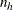denotes the sample size (the number of units in the sample) for stratum h, and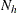denotes the population size (number of units in the population) for stratum h, for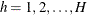. When the sample design is not stratified, n denotes the sample size, and N denotes the population size. For PPS sampling,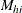represents the size measure for unit i in stratum h,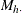is the total of all size measures for the population of stratum h, and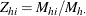is the relative size of unit i in stratum h.